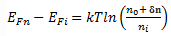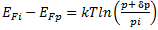Courses

# Test: Fermi Level In A Semiconductor Having Impurities

## 10 Questions MCQ Test Electronic Devices | Test: Fermi Level In A Semiconductor Having Impurities

Description
This mock test of Test: Fermi Level In A Semiconductor Having Impurities for Electrical Engineering (EE) helps you for every Electrical Engineering (EE) entrance exam. This contains 10 Multiple Choice Questions for Electrical Engineering (EE) Test: Fermi Level In A Semiconductor Having Impurities (mcq) to study with solutions a complete question bank. The solved questions answers in this Test: Fermi Level In A Semiconductor Having Impurities quiz give you a good mix of easy questions and tough questions. Electrical Engineering (EE) students definitely take this Test: Fermi Level In A Semiconductor Having Impurities exercise for a better result in the exam. You can find other Test: Fermi Level In A Semiconductor Having Impurities extra questions, long questions & short questions for Electrical Engineering (EE) on EduRev as well by searching above.
QUESTION: 1

### Which states get filled in the conduction band when the donor-type impurity is added to a crystal?

Solution:

When the donor-type impurity is added to a crystal, first Nd states get filled because it is of the highest energy.

QUESTION: 2

### Which of the following expression represent the correct formulae for calculating the exact position of the Fermi level for p-type material?

Solution:

The correct position of the Fermi level is found with the formula in the ‘a’ option.

QUESTION: 3

### Where will be the position of the Fermi level of the n-type material when ND=NA?

Solution:

When ND=NA, kTln(ND/NA )=0
So,
Ef=Ec.

QUESTION: 4

When the temperature of either n-type or p-type increases, determine the movement of the position of the Fermi energy level?

Solution:

whenever the temperature increases, the Fermi energy level tends to move at the centre of the energy gap.

QUESTION: 5

Is it true, when the temperature rises, the electrons in the conduction band becomes greater than the donor atoms?

Solution:

When the temperature increases, there is an increase in the electron-hole pairs and all the donor atoms get ionized, so now the thermally generated electrons will be greater than the donor atoms.

QUESTION: 6

If the excess carriers are created in the semiconductor, then identify the correct energy level diagram.

Solution:

The diagram A refers the most suitable energy level diagrams because Efp>Ef>Efi>Efp>Ev.

QUESTION: 7

If excess charge carriers are created in the semiconductor then the new Fermi level is known as Quasi-Fermi level. Is it true?

Solution:

Quasi-fermi level is defined as the change in the level of the Fermi level when the excess chare carriers are added to the semiconductor.

QUESTION: 8

Ef lies in the middle of the energy level indicates the unequal concentration of the holes and the electrons?

Solution:

When the Ef is in the middle of the energy level, it indicates the equal concentration of the holes and electrons.

QUESTION: 9

Consider a bar of silicon having carrier concentration n0=1015 cm-3 and ni=1010cm-3. Assume the excess carrier concentrations to be n=1013cm-3, calculate the quasi-fermi energy level at T=300K?

Solution:=1.38*10-23*300*ln(1013+1015/1013)
=0.2984 eV.

QUESTION: 10

From the above equation, assuming the same values for the for ni, n= p and T. Given that p0=105cm-3. Calculate the quasi-fermi energy level in eV?

Solution:

Using the same equation,Substituting the respective values,
EFi – EFp=0.1792 eV.